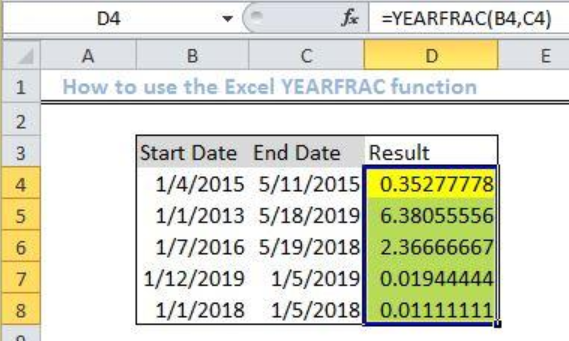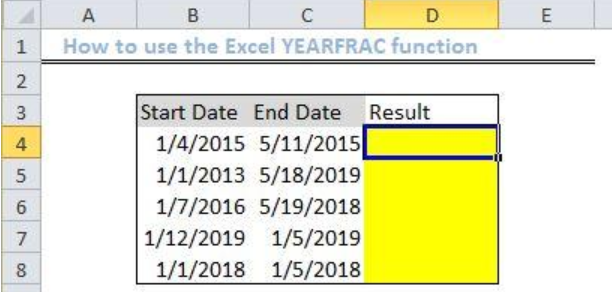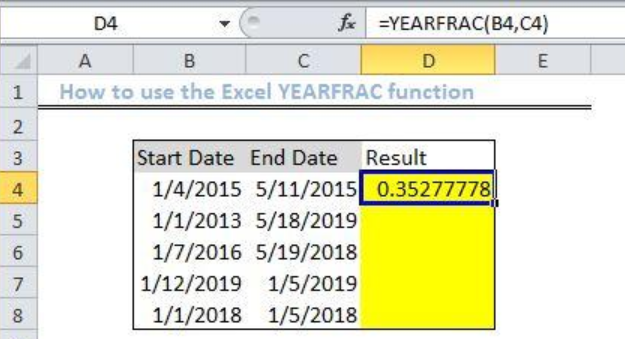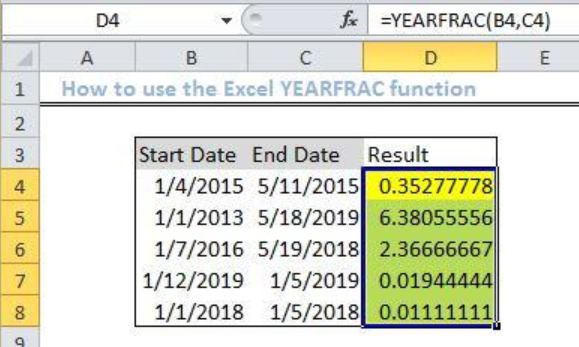Get instant live expert help with Excel or Google Sheets“My Excelchat expert helped me in less than 20 minutes, saving me what would have been 5 hours of work!”

#### Post your problem and you’ll get expert help in seconds.

Your message must be at least 40 characters
Our professional experts are available now. Your privacy is guaranteed.

# Excel YEARFRAC Function

We can use the Excel YEARFRAC function to extract the number of days between two dates as a fraction of a specified year.  We will work through the process of using this function in the simple steps below.Figure 1: Result of the Excel YEARFRAC function

## General Formula

`=YEARFRAC(start_date, end_date, [basis])`

## Formula

`=YEARFRAC(B4,C4)`

## Setting up the Data

• We will set up our data by inputting the Start Date in Column B
• Column C will contain the End date
• Column D is where will we input the formula to derive the resultFigure 2: Setting up the Data

## Applying the YEARFRAC function

• We will click on Cell D4
• We will input the formula below in the cell
`=YEARFRAC(B4,C4)`
• Next, we will press the enter keyFigure 3: Applying the Excel YEARFRAC function

• We click again on Cell D4 and use the fill handle tool (a plus sign found at the base of Cell D4) to drag down the formula into the other cells.Figure 4: Result using the Excel YEARFRAC function

## Explanation

We use the YEARFRAC function to calculate the fractional year between two dates. This function will return with the decimal value which is the fraction of a year between the two periods. We can also use this function to calculate birthdate, retirement dates, number of years between two particular dates, etc.

## Notes:

• If the dates entered are returning with an error, check whether they are introduced as text and change your approach by entering them with the DATE() function.
• If your dates are not valid, then you will have the #VALUE! Error.
• If basis>0 or if basis <4 then YEARFRAC function will return error #NUM!

## Instant Connection to an Expert through our Excelchat Service

Most of the time, the problem you will need to solve will be more complex than a simple application of a formula or function. If you want to save hours of research and frustration, try our live Excelchat service! Our Excel Experts are available 24/7 to answer any Excel question you may have. We guarantee a connection within 30 seconds and a customized solution within 20 minutes.

### Did this post not answer your question? Get a solution from connecting with the expert.Another blog reader asked this question today on Excelchat:
Solution examplesI need assistance setting up a time sheet formula where I can create a column of contiguous dates down the left hand side of the spread sheet. So I can copy a contiguous column of dates, rather than entering each date manually. I would only need to have work days in the list, ie Monday to Friday inc. with a couple of spare lines between each week.
Solved by Z. Y. in 40 minsI have multiple columns on my spread sheet and want to switch from A-Z to Z-A based on values in column A
Solved by V. H. in 38 minsConcat formula is incorrectly formatting the date in column C. Currently column B has dd/mm/yyyy and I require mm/dd/yyyy
Solved by Z. Y. in 31 minsI need a formula that has these 2 rules. If letter was rec'd between 1st and 15th = 1st of the month following letter rec'd date. If letter was rec'd between 16thst and end of the month= 1st of the 2nd month following letter rec'd date.
Solved by V. F. in 20 minsI need a formula that will allow me to calc before a certain date and after a certain date. =if(A2<=DATE(2015,8,27),(F2*1.14),(F2*1.15)) It just multiplies column F with 1.15. I'm assuming I need to bring in the date column into the equation?
Solved by M. Q. in 20 mins## Subscribe to Excelchat.coAnother blog reader asked this question today on Excelchat: